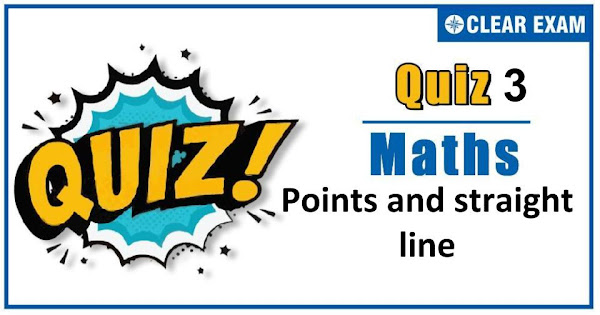## [LATEST]\$type=sticky\$show=home\$rm=0\$va=0\$count=4\$va=0

Dear Readers, As per analysis for previous years, it has been observed that students preparing for JEE MAINS find Mathematics out of all the sections to be complex to handle and the majority of them are not able to comprehend the reason behind it. This problem arises especially because these aspirants appearing for the examination are more inclined to have a keen interest in Mathematics due to their ENGINEERING background.
Furthermore, sections such as Mathematics are dominantly based on theories, laws, numerical in comparison to a section of Engineering which is more of fact-based, Physics, and includes substantial explanations. By using the table given below, you easily and directly access to the topics and respective links of MCQs. Moreover, to make learning smooth and efficient, all the questions come with their supportive solutions to make utilization of time even more productive. Students will be covered for all their studies as the topics are available from basics to even the most advanced.
.

Q1.  The distance between the pair of parallel lines given by x2-1005x+2006=0 is
•  1001
•  1000
•  1005
•  2006
Solution
a) Given equation is x2-1005x+2006=0 ⇒ (x-2)(x-1003)=0 ⇒ x=2,x=1003 ∴ Required distance between the lines =1003-2=1001
Q2. The area of the triangle formed by the axes and the line (cos⁡hα-sin⁡hα )+(cos⁡hα+sin⁡ hα)y=2 in square units, is
•  4
•  3
•   2
•  1
Solution
c) Given line meets the coordinate axes at A(2/cos⁡h α-sin⁡h α ,0)and B(0,2/cos⁡h α+sin⁡h α) ∴Area of ∆ OAB =1/2×OA×OB =1/2×2/cos⁡h α-sin⁡h α ×2/cos⁡h α+sin⁡hα =2/(cos⁡h2α-sin⁡h2α)=2 sq.units
Q3.  The area enclosed within the curve |x|+|y|=1, is
•  1 sq. units
•  2 sq. units
•  3 sq. units
•  4 sq. units
Solution
(b) The given curve is |x|+|y|=1 ⇒x+y=1 for x≥0,y≥0 -x-y=1 for x<0,y<0 x-y=1 for x≥0,y<0 -x+y=1 for x≤0,y≥0 These lines represent a square as shown in Fig.S.5 such that the length of each side is √2 units ∴ Area enclosed =√2×√2=2 sq.units

Q4. The vertices of ∆OBC are (0, 0), (-3,-1) and (-1,-3), then the equation of the line parallel to BC which is a distance 1/2 from the origin and cut OB and OC intercept, is
•  2x-2y+√2=0
•  2x+2y+√2=0
•  2x+2y-√2=0
•  x+y√2=0
Solution
(a) Since, the angle is right angle. ∴Homogenising,x2+y2=4((y-3x)/c)2 ⇒ c2 (x2+y2)=4(y2+9x2-6xy) Since, lines are at right angle. ∴ Coefficient of x2+coefficient of y2=0 ⇒ c2-36+c2-4=0 ⇒ c2=20
Q5. A pair of perpendicular straight lines passes through the origin and also through the point of intersection of the curve
x2+y2=4 with x+y=a. The set containing the value of ‘a’ is
•  {-2,2}
•  {-3,3}
•  {-4,4}
•  {-5,5}
Solution
a) To make the given curves x2+y2=4 and x+y=a homogeneous. x2+y2-4((x+y)/a)2=0 ⇒ a2 (x2+y^2 )-4(x2+y2+2xy)=0 ⇒ x 2(a2-4)+y2 (a2-4)-8xy=0 Since, this is a perpendicular pair of straight lines. ∴ a2-4+a2-4=0 ⇒ a2=4 ⇒ a=±2 Hence, required set of a is {-2,2}.
Q6. The equation of a straight line which passes through the point acos3θ,asin3θ and perpendicular to xsec⁡θ + ycosec θ=a is
•  x/a + y/a=a cos⁡θ
•  xcos⁡θ-ysin⁡θ=-a cos⁡2θ
•  x cos⁡θ+y sin⁡θ=a cos⁡2θ
•   x cos⁡θ+y sin⁡θ-a cos⁡2θ
Solution
b) Required equation of line is y-a sin3 θ=cosecθ/sec⁡θ (x-acos3 θ) ⇒y-a sin3 θ=cosθ/sin⁡θ (x-a cos 3θ) ⇒y sin⁡θ-asin4θ=x cos⁡θ-a cos4 θ ⇒x cos⁡θ-y sin⁡θ+asin4θ-a cos4θ=0 ⇒x cos⁡θ- y sin⁡θ+a cos⁡2 θ=0
Q7.The image of the point (1,3) in the line x+y-6=0, is
•   (3,5)
•  (5,3)
•  (1,-3)
•  (-1,3)
Solution
a) Image of (1,3) in the line x+y-6=0 is given by (x-1)/1=(y-3)/1=-2((1+3-6)/(12+12 ))⇒x=3,y=5 Hence, the image of the given point has coordinates (3,5)
Q8.The angle between the lines √3 x-y-2=0 and x-√3 y+1=0 is
•  90°
•  60°
•  45°
•  30°
Solution
(d) Slopes of given lines are m_1=√3 and m_2=1/√3 ∴ tan⁡θ= |(√3-1/√3)/(1+1)|=|(3-1)/(2√3)|=1/√3 ⇒ θ=30°
Q9. The lines bisecting the angle between the bisectors of the angles between the lines ax2+2hxy+by2 are given by
•  (a-b)(x2-y2)-4 hxy=0
•   (a-b)(x2+y2 )+4hxy=0
•  (a-b)(x2-y2)+4 hxy=0
•  None of these
Solution
(c) The equation representing the bisectors of the angles between the lines given by ax2+2hxy+by2=0 is (x2-y2)/(a-b)=xy/h ⇒hx2-(a-b)xy-hy2=0 …(i) The combined equation of the bisectors of the angles between these lines is (x2-y2)/(h+h)=xy/(-((a-b))/2)⇒(a-b)(x2-y2)+4hxy=0
Q10. The equation of the straight line which is perpendicular to y=x and passes through (3, 2) is
•  x-y=5
•  x+y=5
•  x+y=1
•  x-y=1
Solution
(b) ∵ Slope of given line y=x is 1 ∴ Slope of required line which is perpendicular to given line is -1 Thus, the equation of required line passing through (3, 2) and slope -1, is y-2=-1(x-3) ⇒ x+y=5#### Written by: AUTHORNAME

AUTHORDESCRIPTION## Want to know more

Please fill in the details below:

## Latest NEET Articles\$type=three\$c=3\$author=hide\$comment=hide\$rm=hide\$date=hide\$snippet=hide

Name

ltr
item
BEST NEET COACHING CENTER | BEST IIT JEE COACHING INSTITUTE | BEST NEET, IIT JEE COACHING INSTITUTE: Point and Straight line Quiz-3
Point and Straight line Quiz-3
https://1.bp.blogspot.com/--dbj057FPCU/X6QXweI5eAI/AAAAAAAAKP0/2y-G8jAvSIoFU8JX-8BYWm9Obldb8yIDwCLcBGAsYHQ/s600/Copy%2Bof%2BQuiz%2BImage%2BTemplate%2B%25281%2529%2B%25282%2529.jpg
https://1.bp.blogspot.com/--dbj057FPCU/X6QXweI5eAI/AAAAAAAAKP0/2y-G8jAvSIoFU8JX-8BYWm9Obldb8yIDwCLcBGAsYHQ/s72-c/Copy%2Bof%2BQuiz%2BImage%2BTemplate%2B%25281%2529%2B%25282%2529.jpg
BEST NEET COACHING CENTER | BEST IIT JEE COACHING INSTITUTE | BEST NEET, IIT JEE COACHING INSTITUTE
https://www.cleariitmedical.com/2020/11/point-and-straight-line-quiz-3.html
https://www.cleariitmedical.com/
https://www.cleariitmedical.com/
https://www.cleariitmedical.com/2020/11/point-and-straight-line-quiz-3.html
true
7783647550433378923
UTF-8